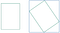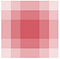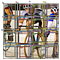# Data Augmentation and Bounding Box [2:58]

`tfms = tfms_from_model(f_model, sz, crop_type=CropType.NO,                        aug_tfms=augs)md = ImageClassifierData.from_csv(PATH, JPEGS, BB_CSV, tfms=tfms,                                  continuous=True, bs=4)`

## Let’s create some data augmentation [4:40]

`augs = [RandomFlip(),         RandomRotate(30),        RandomLighting(0.1,0.1)]`
`tfms = tfms_from_model(f_model, sz, crop_type=CropType.NO,                       aug_tfms=augs)md = ImageClassifierData.from_csv(PATH, JPEGS, BB_CSV, tfms=tfms,                       continuous=True, bs=4)idx=3fig,axes = plt.subplots(3,3, figsize=(9,9))for i,ax in enumerate(axes.flat):    x,y=next(iter(md.aug_dl))    ima=md.val_ds.denorm(to_np(x))[idx]    b = bb_hw(to_np(y[idx]))    print(b)    show_img(ima, ax=ax)    draw_rect(ax, b)[ 115.   63.  240.  311.][ 115.   63.  240.  311.][ 115.   63.  240.  311.][ 115.   63.  240.  311.][ 115.   63.  240.  311.][ 115.   63.  240.  311.][ 115.   63.  240.  311.][ 115.   63.  240.  311.][ 115.   63.  240.  311.]`
`augs = [RandomFlip(tfm_y=TfmType.COORD),        RandomRotate(30, tfm_y=TfmType.COORD),        RandomLighting(0.1,0.1, tfm_y=TfmType.COORD)]tfms = tfms_from_model(f_model, sz, crop_type=CropType.NO,                       tfm_y=TfmType.COORD, aug_tfms=augs)md = ImageClassifierData.from_csv(PATH, JPEGS, BB_CSV, tfms=tfms,                        continuous=True, bs=4)`
`idx=3fig,axes = plt.subplots(3,3, figsize=(9,9))for i,ax in enumerate(axes.flat):    x,y=next(iter(md.aug_dl))    ima=md.val_ds.denorm(to_np(x))[idx]    b = bb_hw(to_np(y[idx]))    print(b)    show_img(ima, ax=ax)    draw_rect(ax, b)[  48.   34.  112.  188.][  65.   36.  107.  185.][  49.   27.  131.  195.][  24.   18.  147.  204.][  61.   34.  113.  188.][  55.   31.  121.  191.][  52.   19.  144.  203.][   7.    0.  193.  222.][  52.   38.  105.  182.]`This why the box gets bigger
`tfm_y = TfmType.COORDaugs = [RandomFlip(tfm_y=tfm_y),        RandomRotate(3, p=0.5, tfm_y=tfm_y),        RandomLighting(0.05,0.05, tfm_y=tfm_y)]tfms = tfms_from_model(f_model, sz, crop_type=CropType.NO,                  tfm_y=tfm_y, aug_tfms=augs)md = ImageClassifierData.from_csv(PATH, JPEGS, BB_CSV, tfms=tfms,                  continuous=True)`

## custom_head [9:34]

`head_reg4 = nn.Sequential(Flatten(), nn.Linear(25088,4))learn = ConvLearner.pretrained(f_model, md, custom_head=head_reg4)learn.opt_fn = optim.Adamlearn.crit = nn.L1Loss()`

## 1. Providing Data

`f_model=resnet34sz=224bs=64val_idxs = get_cv_idxs(len(trn_fns))tfms = tfms_from_model(f_model, sz, crop_type=CropType.NO,                        tfm_y=TfmType.COORD, aug_tfms=augs)md = ImageClassifierData.from_csv(PATH, JPEGS, BB_CSV, tfms=tfms,                        continuous=True, val_idxs=val_idxs)md2 = ImageClassifierData.from_csv(PATH, JPEGS, CSV,                       tfms=tfms_from_model(f_model, sz))`
`class ConcatLblDataset(Dataset):    def __init__(self, ds, y2): self.ds,self.y2 = ds,y2    def __len__(self): return len(self.ds)        def __getitem__(self, i):        x,y = self.ds[i]        return (x, (y,self.y2[i]))`
`trn_ds2 = ConcatLblDataset(md.trn_ds, md2.trn_y)val_ds2 = ConcatLblDataset(md.val_ds, md2.val_y)`
`val_ds2(array([   0.,   49.,  205.,  180.], dtype=float32), 14)`
`md.trn_dl.dataset = trn_ds2md.val_dl.dataset = val_ds2`
`x,y = next(iter(md.val_dl))idx = 3ima = md.val_ds.ds.denorm(to_np(x))[idx]b = bb_hw(to_np(y[idx])); barray([  52.,   38.,  106.,  184.], dtype=float32)ax = show_img(ima)draw_rect(ax, b)draw_text(ax, b[:2], md2.classes[y[idx]])`

## 2. Choosing Architecture [13:54]

`head_reg4 = nn.Sequential(    Flatten(),    nn.ReLU(),    nn.Dropout(0.5),    nn.Linear(25088,256),    nn.ReLU(),    nn.BatchNorm1d(256),    nn.Dropout(0.5),    nn.Linear(256,4+len(cats)),)models = ConvnetBuilder(f_model, 0, 0, 0, custom_head=head_reg4)learn = ConvLearner(md, models)learn.opt_fn = optim.Adam`

## 3. Loss Function [15:46]

`def detn_loss(input, target):    bb_t,c_t = target    bb_i,c_i = input[:, :4], input[:, 4:]    bb_i = F.sigmoid(bb_i)*224    # I looked at these quantities separately first then picked a     # multiplier to make them approximately equal    return F.l1_loss(bb_i, bb_t) + F.cross_entropy(c_i, c_t)*20def detn_l1(input, target):    bb_t,_ = target    bb_i = input[:, :4]    bb_i = F.sigmoid(bb_i)*224    return F.l1_loss(V(bb_i),V(bb_t)).datadef detn_acc(input, target):    _,c_t = target    c_i = input[:, 4:]    return accuracy(c_i, c_t)learn.crit = detn_losslearn.metrics = [detn_acc, detn_l1]`
`lr=1e-2learn.fit(lr, 1, cycle_len=3, use_clr=(32,5))epoch      trn_loss   val_loss   detn_acc   detn_l1           0      72.036466  45.186367  0.802133   32.647586     1      51.037587  36.34964   0.828425   25.389733         2      41.4235    35.292709  0.835637   24.343577[35.292709, 0.83563701808452606, 24.343576669692993]`
`learn.save('reg1_0')learn.freeze_to(-2)lrs = np.array([lr/100, lr/10, lr])learn.fit(lrs/5, 1, cycle_len=5, use_clr=(32,10))epoch      trn_loss   val_loss   detn_acc   detn_l1           0      34.448113  35.972973  0.801683   22.918499     1      28.889909  33.010857  0.830379   21.689888         2      24.237017  30.977512  0.81881    20.817996         3      21.132993  30.60677   0.83143    20.138552         4      18.622983  30.54178   0.825571   19.832196[30.54178, 0.82557091116905212, 19.832195997238159]learn.unfreeze()learn.fit(lrs/10, 1, cycle_len=10, use_clr=(32,10))epoch      trn_loss   val_loss   detn_acc   detn_l1           0      15.957164  31.111507  0.811448   19.970753     1      15.955259  32.597153  0.81235    20.111022         2      15.648723  32.231941  0.804087   19.522853         3      14.876172  30.93821   0.815805   19.226574         4      14.113872  31.03952   0.808594   19.155093         5      13.293885  29.736671  0.826022   18.761728         6      12.562566  30.000023  0.827524   18.82006          7      11.885125  30.28841   0.82512    18.904158         8      11.498326  30.070133  0.819712   18.635296         9      11.015841  30.213772  0.815805   18.551489[30.213772, 0.81580528616905212, 18.551488876342773]`

# Multi label classification [25:29]

`%matplotlib inline%reload_ext autoreload%autoreload 2from fastai.conv_learner import *from fastai.dataset import *import json, pdbfrom PIL import ImageDraw, ImageFontfrom matplotlib import patches, patheffectstorch.backends.cudnn.benchmark=True`

## Setup

`PATH = Path('data/pascal')trn_j = json.load((PATH / 'pascal_train2007.json').open())IMAGES,ANNOTATIONS,CATEGORIES = ['images', 'annotations',                                  'categories']FILE_NAME,ID,IMG_ID,CAT_ID,BBOX = 'file_name','id','image_id',                                   'category_id','bbox'cats = dict((o[ID], o['name']) for o in trn_j[CATEGORIES])trn_fns = dict((o[ID], o[FILE_NAME]) for o in trn_j[IMAGES])trn_ids = [o[ID] for o in trn_j[IMAGES]]JPEGS = 'VOCdevkit/VOC2007/JPEGImages'IMG_PATH = PATH/JPEGSdef get_trn_anno():    trn_anno = collections.defaultdict(lambda:[])    for o in trn_j[ANNOTATIONS]:        if not o['ignore']:            bb = o[BBOX]            bb = np.array([bb, bb, bb+bb-1,                            bb+bb-1])            trn_anno[o[IMG_ID]].append((bb,o[CAT_ID]))    return trn_annotrn_anno = get_trn_anno()def show_img(im, figsize=None, ax=None):    if not ax: fig,ax = plt.subplots(figsize=figsize)    ax.imshow(im)    ax.set_xticks(np.linspace(0, 224, 8))    ax.set_yticks(np.linspace(0, 224, 8))    ax.grid()    ax.set_yticklabels([])    ax.set_xticklabels([])    return axdef draw_outline(o, lw):    o.set_path_effects([patheffects.Stroke(        linewidth=lw, foreground='black'), patheffects.Normal()])def draw_rect(ax, b, color='white'):    patch = ax.add_patch(patches.Rectangle(b[:2], *b[-2:],                          fill=False, edgecolor=color, lw=2))    draw_outline(patch, 4)def draw_text(ax, xy, txt, sz=14, color='white'):    text = ax.text(*xy, txt,        verticalalignment='top', color=color, fontsize=sz,         weight='bold')    draw_outline(text, 1)def bb_hw(a): return np.array([a,a,a-a,a-a])def draw_im(im, ann):    ax = show_img(im, figsize=(16,8))    for b,c in ann:        b = bb_hw(b)        draw_rect(ax, b)        draw_text(ax, b[:2], cats[c], sz=16)def draw_idx(i):    im_a = trn_anno[i]    im = open_image(IMG_PATH/trn_fns[i])    draw_im(im, im_a)`

## Multi class [26:12]

`MC_CSV = PATH/'tmp/mc.csv'trn_anno[(array([ 96, 155, 269, 350]), 7)]mc = [set([cats[p] for p in trn_anno[o]]) for o in trn_ids]mcs = [' '.join(str(p) for p in o) for o in mc]df = pd.DataFrame({'fn': [trn_fns[o] for o in trn_ids],                    'clas': mcs}, columns=['fn','clas'])df.to_csv(MC_CSV, index=False)`
`f_model=resnet34sz=224bs=64tfms = tfms_from_model(f_model, sz, crop_type=CropType.NO)md = ImageClassifierData.from_csv(PATH, JPEGS, MC_CSV, tfms=tfms)learn = ConvLearner.pretrained(f_model, md)learn.opt_fn = optim.Adamlr = 2e-2learn.fit(lr, 1, cycle_len=3, use_clr=(32,5))epoch      trn_loss   val_loss   <lambda>                      0      0.104836   0.085015   0.972356      1      0.088193   0.079739   0.972461                       2      0.072346   0.077259   0.974114[0.077258907, 0.9741135761141777]lrs = np.array([lr/100, lr/10, lr])learn.freeze_to(-2)learn.fit(lrs/10, 1, cycle_len=5, use_clr=(32,5))epoch      trn_loss   val_loss   <lambda>                       0      0.063236   0.088847   0.970681      1      0.049675   0.079885   0.973723                       2      0.03693    0.076906   0.975601                       3      0.026645   0.075304   0.976187                       4      0.018805   0.074934   0.975165[0.074934497, 0.97516526281833649]learn.save('mclas')learn.load('mclas')y = learn.predict()x,_ = next(iter(md.val_dl))x = to_np(x)fig, axes = plt.subplots(3, 4, figsize=(12, 8))for i,ax in enumerate(axes.flat):    ima=md.val_ds.denorm(x)[i]    ya = np.nonzero(y[i]>0.4)    b = '\n'.join(md.classes[o] for o in ya)    ax = show_img(ima, ax=ax)    draw_text(ax, (0,0), b)plt.tight_layout()`
`mc = [set([cats[p] for p in trn_anno[o]]) for o in trn_ids]`

## Receptive Field [37:20]3x3 convolution with opacity 15% — clearly the center of the box has more dependencies

## Architecture [41:18]

`class StdConv(nn.Module):    def __init__(self, nin, nout, stride=2, drop=0.1):        super().__init__()        self.conv = nn.Conv2d(nin, nout, 3, stride=stride,                               padding=1)        self.bn = nn.BatchNorm2d(nout)        self.drop = nn.Dropout(drop)            def forward(self, x):         return self.drop(self.bn(F.relu(self.conv(x))))        def flatten_conv(x,k):    bs,nf,gx,gy = x.size()    x = x.permute(0,2,3,1).contiguous()    return x.view(bs,-1,nf//k)class OutConv(nn.Module):    def __init__(self, k, nin, bias):        super().__init__()        self.k = k        self.oconv1 = nn.Conv2d(nin, (len(id2cat)+1)*k, 3,                                 padding=1)        self.oconv2 = nn.Conv2d(nin, 4*k, 3, padding=1)        self.oconv1.bias.data.zero_().add_(bias)            def forward(self, x):        return [flatten_conv(self.oconv1(x), self.k),                flatten_conv(self.oconv2(x), self.k)]class SSD_Head(nn.Module):    def __init__(self, k, bias):        super().__init__()        self.drop = nn.Dropout(0.25)        self.sconv0 = StdConv(512,256, stride=1)        self.sconv2 = StdConv(256,256)        self.out = OutConv(k, 256, bias)            def forward(self, x):        x = self.drop(F.relu(x))        x = self.sconv0(x)        x = self.sconv2(x)        return self.out(x)head_reg4 = SSD_Head(k, -3.)models = ConvnetBuilder(f_model, 0, 0, 0, custom_head=head_reg4)learn = ConvLearner(md, models)learn.opt_fn = optim.Adam`

## Testing [51:58]

`x,y = next(iter(md.val_dl))x,y = V(x),V(y)learn.model.eval()batch = learn.model(x)b_clas,b_bb = batchb_clas.size(),b_bb.size()(torch.Size([64, 16, 21]), torch.Size([64, 16, 4]))`
`idx=7b_clasi = b_clas[idx]b_bboxi = b_bb[idx]ima=md.val_ds.ds.denorm(to_np(x))[idx]bbox,clas = get_y(y[idx], y[idx])bbox,clas(Variable containing:  0.6786  0.4866  0.9911  0.6250  0.7098  0.0848  0.9911  0.5491  0.5134  0.8304  0.6696  0.9063 [torch.cuda.FloatTensor of size 3x4 (GPU 0)], Variable containing:   8  10  17 [torch.cuda.LongTensor of size 3 (GPU 0)])`
`def torch_gt(ax, ima, bbox, clas, prs=None, thresh=0.4):    return show_ground_truth(ax, ima, to_np((bbox*224).long()),         to_np(clas),          to_np(prs) if prs is not None else None, thresh)fig, ax = plt.subplots(figsize=(7,7))torch_gt(ax, ima, bbox, clas)`
`fig, ax = plt.subplots(figsize=(7,7))torch_gt(ax, ima, anchor_cnr, b_clasi.max(1))`
`anchorsVariable containing: 0.1250  0.1250  0.2500  0.2500 0.1250  0.3750  0.2500  0.2500 0.1250  0.6250  0.2500  0.2500 0.1250  0.8750  0.2500  0.2500 0.3750  0.1250  0.2500  0.2500 0.3750  0.3750  0.2500  0.2500 0.3750  0.6250  0.2500  0.2500 0.3750  0.8750  0.2500  0.2500 0.6250  0.1250  0.2500  0.2500 0.6250  0.3750  0.2500  0.2500 0.6250  0.6250  0.2500  0.2500 0.6250  0.8750  0.2500  0.2500 0.8750  0.1250  0.2500  0.2500 0.8750  0.3750  0.2500  0.2500 0.8750  0.6250  0.2500  0.2500 0.8750  0.8750  0.2500  0.2500[torch.cuda.FloatTensor of size 16x4 (GPU 0)]`
`overlaps = jaccard(bbox.data, anchor_cnr.data)overlapsColumns 0 to 7   0.0000  0.0000  0.0000  0.0000  0.0000  0.0000  0.0000  0.0000    0.0000  0.0000  0.0000  0.0000  0.0000  0.0000  0.0000  0.0000    0.0000  0.0000  0.0000  0.0000  0.0000  0.0000  0.0000  0.0000      Columns 8 to 15   0.0000  0.0091 0.0922  0.0000  0.0000  0.0315  0.3985  0.0000  0.0356  0.0549 0.0103  0.0000  0.2598  0.4538  0.0653  0.0000  0.0000  0.0000 0.0000  0.1897  0.0000  0.0000  0.0000  0.0000 [torch.cuda.FloatTensor of size 3x16 (GPU 0)]`
`overlaps.max(1)(  0.3985  0.4538  0.1897 [torch.cuda.FloatTensor of size 3 (GPU 0)],   14  13  11 [torch.cuda.LongTensor of size 3 (GPU 0)])`
`overlaps.max(0)(  0.0000  0.0000  0.0000  0.0000  0.0000  0.0000  0.0000  0.0000  0.0356  0.0549  0.0922  0.1897  0.2598  0.4538  0.3985  0.0000 [torch.cuda.FloatTensor of size 16 (GPU 0)],   0  0  0  0  0  0  0  0  1  1  0  2  1  1  0  0 [torch.cuda.LongTensor of size 16 (GPU 0)])`
`gt_overlap,gt_idx = map_to_ground_truth(overlaps)gt_overlap,gt_idx(  0.0000  0.0000  0.0000  0.0000  0.0000  0.0000  0.0000  0.0000  0.0356  0.0549  0.0922  1.9900  0.2598  1.9900  1.9900  0.0000 [torch.cuda.FloatTensor of size 16 (GPU 0)],   0  0  0  0  0  0  0  0  1  1  0  2  1  1  0  0 [torch.cuda.LongTensor of size 16 (GPU 0)])`
`gt_clas = clas[gt_idx]; gt_clasVariable containing:  8  8  8  8  8  8  8  8 10 10  8 17 10 10  8  8[torch.cuda.LongTensor of size 16 (GPU 0)]`
`thresh = 0.5pos = gt_overlap > threshpos_idx = torch.nonzero(pos)[:,0]neg_idx = torch.nonzero(1-pos)[:,0]pos_idx 11 13 14[torch.cuda.LongTensor of size 3 (GPU 0)]`
`gt_clas[1-pos] = len(id2cat)[id2cat[o] if o<len(id2cat) else 'bg' for o in gt_clas.data]['bg', 'bg', 'bg', 'bg', 'bg', 'bg', 'bg', 'bg', 'bg', 'bg', 'bg', 'sofa', 'bg', 'diningtable', 'chair', 'bg']`
`gt_bbox = bbox[gt_idx]loc_loss = ((a_ic[pos_idx] - gt_bbox[pos_idx]).abs()).mean()clas_loss  = F.cross_entropy(b_clasi, gt_clas)loc_loss,clas_loss(Variable containing: 1.00000e-02 *   6.5887 [torch.cuda.FloatTensor of size 1 (GPU 0)], Variable containing:  1.0331 [torch.cuda.FloatTensor of size 1 (GPU 0)])`
`fig, axes = plt.subplots(3, 4, figsize=(16, 12))for idx,ax in enumerate(axes.flat):    ima=md.val_ds.ds.denorm(to_np(x))[idx]    bbox,clas = get_y(y[idx], y[idx])    ima=md.val_ds.ds.denorm(to_np(x))[idx]    bbox,clas = get_y(bbox,clas); bbox,clas    a_ic = actn_to_bb(b_bb[idx], anchors)    torch_gt(ax, ima, a_ic, b_clas[idx].max(1),              b_clas[idx].max(1).sigmoid(), 0.01)plt.tight_layout()`

## Tweak 1. How do we interpret the activations [1:04:16]?

`def actn_to_bb(actn, anchors):    actn_bbs = torch.tanh(actn)    actn_centers = (actn_bbs[:,:2]/2 * grid_sizes) + anchors[:,:2]    actn_hw = (actn_bbs[:,2:]/2+1) * anchors[:,2:]    return hw2corners(actn_centers, actn_hw)`

## Tweak 2. We actually use binary cross entropy loss instead of cross entropy [1:05:36]

`class BCE_Loss(nn.Module):    def __init__(self, num_classes):        super().__init__()        self.num_classes = num_classes    def forward(self, pred, targ):        t = one_hot_embedding(targ, self.num_classes+1)        t = V(t[:,:-1].contiguous())#.cpu()        x = pred[:,:-1]        w = self.get_weight(x,t)        return F.binary_cross_entropy_with_logits(x, t, w,                             size_average=False)/self.num_classes        def get_weight(self,x,t): return None`

## SSD Loss Function [1:09:55]

`def ssd_1_loss(b_c,b_bb,bbox,clas,print_it=False):    bbox,clas = get_y(bbox,clas)    a_ic = actn_to_bb(b_bb, anchors)    overlaps = jaccard(bbox.data, anchor_cnr.data)    gt_overlap,gt_idx = map_to_ground_truth(overlaps,print_it)    gt_clas = clas[gt_idx]    pos = gt_overlap > 0.4    pos_idx = torch.nonzero(pos)[:,0]    gt_clas[1-pos] = len(id2cat)    gt_bbox = bbox[gt_idx]    loc_loss = ((a_ic[pos_idx] - gt_bbox[pos_idx]).abs()).mean()    clas_loss  = loss_f(b_c, gt_clas)    return loc_loss, clas_lossdef ssd_loss(pred,targ,print_it=False):    lcs,lls = 0.,0.    for b_c,b_bb,bbox,clas in zip(*pred,*targ):        loc_loss,clas_loss = ssd_1_loss(b_c,b_bb,bbox,clas,print_it)        lls += loc_loss        lcs += clas_loss    if print_it: print(f'loc: {lls.data}, clas: {lcs.data}')    return lls+lcs`
`def get_y(bbox,clas):    bbox = bbox.view(-1,4)/sz    bb_keep = ((bbox[:,2]-bbox[:,0])>0).nonzero()[:,0]    return bbox[bb_keep],clas[bb_keep]`

## Training [1:12:47]

`learn.crit = ssd_losslr = 3e-3lrs = np.array([lr/100,lr/10,lr])learn.lr_find(lrs/1000,1.)learn.sched.plot(1)epoch      trn_loss   val_loss                                0      44.232681  21476.816406`
`learn.lr_find(lrs/1000,1.)learn.sched.plot(1)epoch      trn_loss   val_loss                                0      86.852668  32587.789062`
`learn.fit(lr, 1, cycle_len=5, use_clr=(20,10))epoch      trn_loss   val_loss                                0      45.570843  37.099854     1      37.165911  32.165031                               2      33.27844   30.990122                               3      31.12054   29.804482                               4      29.305789  28.943184[28.943184]learn.fit(lr, 1, cycle_len=5, use_clr=(20,10))epoch      trn_loss   val_loss                                0      43.726979  33.803085     1      34.771754  29.012939                               2      30.591864  27.132868                               3      27.896905  26.151638                               4      25.907382  25.739273[25.739273]learn.save('0')learn.load('0')`

## More anchors! [1:14:47]From left (1x1, 2x2, 4x4 grids of anchor boxes). Notice that some of the anchor box is bigger than the original image.
`anc_grids = [4, 2, 1]anc_zooms = [0.75, 1., 1.3]anc_ratios = [(1., 1.), (1., 0.5), (0.5, 1.)]anchor_scales = [(anz*i,anz*j) for anz in anc_zooms                                     for (i,j) in anc_ratios]k = len(anchor_scales)anc_offsets = [1/(o*2) for o in anc_grids]anc_x = np.concatenate([np.repeat(np.linspace(ao, 1-ao, ag), ag)                        for ao,ag in zip(anc_offsets,anc_grids)])anc_y = np.concatenate([np.tile(np.linspace(ao, 1-ao, ag), ag)                        for ao,ag in zip(anc_offsets,anc_grids)])anc_ctrs = np.repeat(np.stack([anc_x,anc_y], axis=1), k, axis=0)anc_sizes = np.concatenate([np.array([[o/ag,p/ag]               for i in range(ag*ag) for o,p in anchor_scales])                 for ag in anc_grids])grid_sizes = V(np.concatenate([np.array([ 1/ag               for i in range(ag*ag) for o,p in anchor_scales])                  for ag in anc_grids]),                       requires_grad=False).unsqueeze(1)anchors = V(np.concatenate([anc_ctrs, anc_sizes], axis=1),               requires_grad=False).float()anchor_cnr = hw2corners(anchors[:,:2], anchors[:,2:])`

## Model Architecture [1:31:10]

`drop=0.4class SSD_MultiHead(nn.Module):    def __init__(self, k, bias):        super().__init__()        self.drop = nn.Dropout(drop)        self.sconv0 = StdConv(512,256, stride=1, drop=drop)        self.sconv1 = StdConv(256,256, drop=drop)        self.sconv2 = StdConv(256,256, drop=drop)        self.sconv3 = StdConv(256,256, drop=drop)        self.out1 = OutConv(k, 256, bias)        self.out2 = OutConv(k, 256, bias)        self.out3 = OutConv(k, 256, bias)    def forward(self, x):        x = self.drop(F.relu(x))        x = self.sconv0(x)        x = self.sconv1(x)        o1c,o1l = self.out1(x)        x = self.sconv2(x)        o2c,o2l = self.out2(x)        x = self.sconv3(x)        o3c,o3l = self.out3(x)        return [torch.cat([o1c,o2c,o3c], dim=1),                torch.cat([o1l,o2l,o3l], dim=1)]head_reg4 = SSD_MultiHead(k, -4.)models = ConvnetBuilder(f_model, 0, 0, 0, custom_head=head_reg4)learn = ConvLearner(md, models)learn.opt_fn = optim.Adam`

## Training [1:32:50]

`learn.crit = ssd_losslr = 1e-2lrs = np.array([lr/100,lr/10,lr])learn.lr_find(lrs/1000,1.)learn.sched.plot(n_skip_end=2)`
`learn.fit(lrs, 1, cycle_len=4, use_clr=(20,8))epoch      trn_loss   val_loss                                0      15.124349  15.015433     1      13.091956  10.39855                                2      11.643629  9.4289                                  3      10.532467  8.822998[8.822998]learn.save('tmp')learn.freeze_to(-2)learn.fit(lrs/2, 1, cycle_len=4, use_clr=(20,8))epoch      trn_loss   val_loss                                0      9.821056   10.335152     1      9.419633   11.834093                               2      8.78818    7.907762                                3      8.219976   7.456364[7.4563637]x,y = next(iter(md.val_dl))y = V(y)batch = learn.model(V(x))b_clas,b_bb = batchx = to_np(x)fig, axes = plt.subplots(3, 4, figsize=(16, 12))for idx,ax in enumerate(axes.flat):    ima=md.val_ds.ds.denorm(x)[idx]    bbox,clas = get_y(y[idx], y[idx])    a_ic = actn_to_bb(b_bb[idx], anchors)    torch_gt(ax, ima, a_ic, b_clas[idx].max(1),              b_clas[idx].max(1).sigmoid(), 0.2)plt.tight_layout()`

# History of object detection [1:33:43]

## Implementing Focal Loss [1:49:27]:

`class BCE_Loss(nn.Module):    def __init__(self, num_classes):        super().__init__()        self.num_classes = num_classes    def forward(self, pred, targ):        t = one_hot_embedding(targ, self.num_classes+1)        t = V(t[:,:-1].contiguous())#.cpu()        x = pred[:,:-1]        w = self.get_weight(x,t)        return F.binary_cross_entropy_with_logits(x, t, w,                           size_average=False)/self.num_classes        def get_weight(self,x,t): return None`
`class FocalLoss(BCE_Loss):    def get_weight(self,x,t):        alpha,gamma = 0.25,2.        p = x.sigmoid()        pt = p*t + (1-p)*(1-t)        w = alpha*t + (1-alpha)*(1-t)        return w * (1-pt).pow(gamma)`

## Training [1:51:25]

`learn.lr_find(lrs/1000,1.)learn.sched.plot(n_skip_end=2)`
`learn.fit(lrs, 1, cycle_len=10, use_clr=(20,10))epoch      trn_loss   val_loss                                0      24.263046  28.975235     1      20.459562  16.362392                               2      17.880827  14.884829                               3      15.956896  13.676485                               4      14.521345  13.134197                               5      13.460941  12.594139                               6      12.651842  12.069849                               7      11.944972  11.956457                               8      11.385798  11.561226                               9      10.988802  11.362164[11.362164]learn.save('fl0')learn.load('fl0')learn.freeze_to(-2)learn.fit(lrs/4, 1, cycle_len=10, use_clr=(20,10))epoch      trn_loss   val_loss                                0      10.871668  11.615532     1      10.908461  11.604334                               2      10.549796  11.486127                               3      10.130961  11.088478                               4      9.70691    10.72144                                5      9.319202   10.600481                               6      8.916653   10.358334                               7      8.579452   10.624706                               8      8.274838   10.163422                               9      7.994316   10.108068[10.108068]learn.save('drop4')learn.load('drop4')plot_results(0.75)`

## Non Maximum Suppression [1:52:15]

`def nms(boxes, scores, overlap=0.5, top_k=100):    keep = scores.new(scores.size(0)).zero_().long()    if boxes.numel() == 0: return keep    x1 = boxes[:, 0]    y1 = boxes[:, 1]    x2 = boxes[:, 2]    y2 = boxes[:, 3]    area = torch.mul(x2 - x1, y2 - y1)    v, idx = scores.sort(0)  # sort in ascending order    idx = idx[-top_k:]  # indices of the top-k largest vals    xx1 = boxes.new()    yy1 = boxes.new()    xx2 = boxes.new()    yy2 = boxes.new()    w = boxes.new()    h = boxes.new()    count = 0    while idx.numel() > 0:        i = idx[-1]  # index of current largest val        keep[count] = i        count += 1        if idx.size(0) == 1: break        idx = idx[:-1]  # remove kept element from view        # load bboxes of next highest vals        torch.index_select(x1, 0, idx, out=xx1)        torch.index_select(y1, 0, idx, out=yy1)        torch.index_select(x2, 0, idx, out=xx2)        torch.index_select(y2, 0, idx, out=yy2)        # store element-wise max with next highest score        xx1 = torch.clamp(xx1, min=x1[i])        yy1 = torch.clamp(yy1, min=y1[i])        xx2 = torch.clamp(xx2, max=x2[i])        yy2 = torch.clamp(yy2, max=y2[i])        w.resize_as_(xx2)        h.resize_as_(yy2)        w = xx2 - xx1        h = yy2 - yy1        # check sizes of xx1 and xx2.. after each iteration        w = torch.clamp(w, min=0.0)        h = torch.clamp(h, min=0.0)        inter = w*h        # IoU = i / (area(a) + area(b) - i)        rem_areas = torch.index_select(area, 0, idx)          # load remaining areas)        union = (rem_areas - inter) + area[i]        IoU = inter/union  # store result in iou        # keep only elements with an IoU <= overlap        idx = idx[IoU.le(overlap)]    return keep, countdef show_nmf(idx):    ima=md.val_ds.ds.denorm(x)[idx]    bbox,clas = get_y(y[idx], y[idx])    a_ic = actn_to_bb(b_bb[idx], anchors)    clas_pr, clas_ids = b_clas[idx].max(1)    clas_pr = clas_pr.sigmoid()    conf_scores = b_clas[idx].sigmoid().t().data    out1,out2,cc = [],[],[]    for cl in range(0, len(conf_scores)-1):        c_mask = conf_scores[cl] > 0.25        if c_mask.sum() == 0: continue        scores = conf_scores[cl][c_mask]        l_mask = c_mask.unsqueeze(1).expand_as(a_ic)        boxes = a_ic[l_mask].view(-1, 4)        ids, count = nms(boxes.data, scores, 0.4, 50)        ids = ids[:count]        out1.append(scores[ids])        out2.append(boxes.data[ids])        cc.append([cl]*count)    cc = T(np.concatenate(cc))    out1 = torch.cat(out1)    out2 = torch.cat(out2)    fig, ax = plt.subplots(figsize=(8,8))    torch_gt(ax, ima, out2, cc, out1, 0.1)for i in range(12): show_nmf(i)`

## Some paper tips [2:02:34]

Welcome to a place where words matter. On Medium, smart voices and original ideas take center stage - with no ads in sight. Watch
Follow all the topics you care about, and we’ll deliver the best stories for you to your homepage and inbox. Explore
Get unlimited access to the best stories on Medium — and support writers while you’re at it. Just \$5/month. Upgrade